## Search This Blog

### 200 versus 200

From our earlier reduction it should be evident that we don't need all twelve counts to uniquely label a scale autocorrelation. Precisely because moving anticlockwise must recover the same autocorrelation result, and because this is true of both non-symmetric and symmetric polygons (which is to say all of them), the number of intervals of size 11 will always be the same as the number of size 1 and - similarly - the numbers for intervals of size 10 and 2, of 9 and 3, of 8 and 4 and of 7 and 5 will always be the same. You may wish to at least verify that statement by looking at the bar charts for the three examples we have thus far demonstrated. The zeroth bar will always be the number of notes in the scale (the number of correlating intervals of size 0) and the remaining eleven bars show a symmetry around their central bar (which counts intervals of size 6).

Thus in the same way that we have twelve distinct pitch classes, identifying the (up to) 12 possible 'notes' in any given scale (i.e. the integers 0 to 11) we have six distinct interval classes identified with the integers 1 to 6 to represent all possible distances between (non-identical) pitch classes.

This means that we could relabel all of the autocorrelations found thus far by dropping the final five digits (for example 725436263452 → 7254362, 733633633633 → 7336336, 623234243232 → 6232342, 521232023212 → 5212320), and in fact any we encounter (mutatis mutandis) later. But for reasons which will soon become clear, we would rather be a little more opaque with the relabelling by taking account of three important considerations.

### Autocorrelation As Interval Vector

(1) We'd like to balance the information content of the label. This means that - if we are to treat - for example - the minor third interval as being counted the same as the major sixth (interval class 3) then rather than mere elision of that major sixth count (by omitting the 9th character of the label) we should add its value to the minor third's appearance (in the fourth character position). This effectively means that the second through sixth characters of the label (counting the ii, II, iii, III and IV intervals, or interval classes 1 to 5) should be doubled. We'd leave the next character alone since the tritone (interval class 6), uniquely, is counted only once. However, and for that very reason, we note that the tritone count will always be an even number since every time this 'six o'clock' vertex is counted clockwisely forwards, there's a corresponding tally anticlockwise. Therefore instead of doubling up the second through sixth digits (and disposing of the eighth through twelfth), it's easier to keep them as they are and simply halve the (always even) seventh digit, which will then become the final character in the label.

What we have actually done here, by dropping the final five counts of the autocorrelation value and halving the (now) final, sixth, count (excluding the 'unison' count right at the beginning) is to count the interval classes rather than the intervals. The six digit (rarely employing A, B, C for 10, 11, 12) string comprising the 2nd to 7th ('halved') characters of our original autocorrelation label is none other than Forte's Interval Vector.

### Autocorrelation Implies Unison

(2) No information is lost if we dispense with the first digit. It's certainly convenient since it tells us instantly and clearly how many notes are in the scale but - although its loss may be felt - the benefits of dispensing with it outweigh (as will be seen next) those of preserving it. In any case, the number of notes in the scale will remain available - though somewhat indirectly - by summing the digits in this now reduced six character label.

### The Musicality of Scales or Chords

(3) Having reduced the autocorrelation label to a six level proportional representation of intervals of ii (minor second), II (major second), iii (minor third), III (major third), IV (perfect fourth) and v (augmented fourth or diminished fifth) on the understanding that the VII, vii, VI, vi and V intervals are also (both respectively and) implicitly being counted thereby, we observe that we're under no obligation to present the counts in that order.

In fact it turns out to be much more musically useful to present them in the order that would result from performing the autocorrelation computation by jumping seven 'hours' at a time rather than just the one. This is merely the cycle of fifths (or fourths) and 12 seven-steps (or 12 five-steps) will (modulo 12) take exactly as long to perform and count precisely the same interval frequencies. I.e. instead of (as in the original) counting intervals in the size order (0, 1, 2, 3, 4, 5, 6, 7, 8, 9, 10, 11) we count them in a modified order (7, 2, 9, 4, 11, 6, 1, 8, 3, 10, 5, 0) from (7, 14, 21, 28, 35, 49, 56, 63, 70, 77, 84) modulo 12. Note that a 12 character label formed from this would still be symmetrical - the final character (where the 0 is) would convey the number of notes in the scale, and the interval equivalences (i.e. 7-5, 2-10, 9-3, 4-8, 11-1 and 6) flow forwards from the 1st character in parallel with a backward flow from the 11th converging to the tritone count at the 6th character.

To perform the 12 to 6 reduction is slightly simpler than one might expect (due to the symmetry in the frequencies of the interval classes). Delete the first character. Delete the last six characters. Swap the (now) first and fifth characters, halve the value of the sixth (now last) character. And we're done.

Ordered in this way, the interval vector is shuffled so that all of the musical consonances turn up, in order, left to right. I.e the most consonant interval, the perfect fourth (or fifth - the interval class is the same) is leftmost, followed by its most consonant interval etc. This would mean that our labels (725436263452, 733633633633, 623234243232, 521232023212), or interval vectors - in the standard notation - (<254361>, <336333>, <232341>, <212320>) will map, one for one, onto new labels (654321, 336333, 432321, 212320). Consonant fifths and thirds etc on the left, moving into minor seconds and tritones on the right.

It was earlier promised that we would still be able to tell from these reduced labels how many notes are in the scale by summing the digits in the label. As promised, we see that both 6+5+4+3+2+1 and 3+3+6+3+3+3 = 21. When the digits sum to 21 we know we have a heptatonic scale. When they sum to 15 we have a hexatonic. When they sum to 10, a pentatonic. Here's another table:

# notes
in scale
label digit
sum
# distinct
labels
lowest
label
highest
label
Dodecatonic Extrema
12 66 1   CCCCC6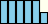Undecatonic Extrema
11 55 1   AAAAA5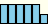Decatonic Extrema
10 45 6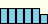888885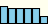988884
Nonatonic Extrema
9 36 12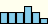666963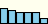876663
Octatonic Extrema
8 28 29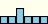448444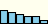765442
Heptatonic Extrema
7 21 36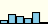254361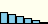654321
Hexatonic Extrema
6 15 35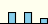060603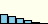543210
Pentatonic Extrema
5 10 36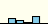032140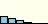432100
Tetratonic Extrema
4 6 29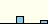004002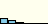321000
Tritonic Extrema
3 3 12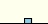000300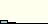210000
Duotonic Extrema
2 1 6000001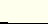100000
Unatonic Extrema
1 0 1   000000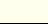The numbers in the label digit sum column are true for all scale sizes and autocorrelations. They are all half of the value which would be obtained by adding the actual interval frequencies. i.e the interval class frequencies. Each value is, for each scale size N, the number of ways of combining pairs of vertices, NC2 = N!/2!(N-2)! = N(N-1)/2.

The mini bar-charts for each case show - as described above - the number of perfect fifths (or perfect fourths - they're the same interval class), then the number of fifths of fifth, fifths of fifths of fifths, etc (up to six) available to a user of the scale in question.

This reordering of the interval vector allows us to 'sort' the scales in descending order and is the basis for the contentious claim that - for each scale order (12 down to 1) we can shake out the 'most consonant' scales to the top of a list and the 'least consonant' scales fall to the bottom of the list.

How much 'natural beauty' one might wish to accord such an ordering is of course completely up to the individual human being. But the sort order is, nevertheless, a real, calculable, property of a system of mathematical objects built purely geometrically from polygons and how far apart their vertices are. The 'musical consonance' attributed to these vertex distances is dependent on the harmoniousness implied by intervalic distance as being representative of ratios of frequencies audible to human beings. If the differences between pitches were (say) additive rather than multiplicative then autocorrelation as a still-legitimate piece of signal processing would be rather less musically useful, if meaningful at all.

The following table presents - in the above sense - the 'best' and 'worst' scales. It should come as no surprise that - of the seven note scales - the 654321 creams off the standard western diatonic scale (and all its ancient Greek modes), and - of the five note scales - the 432100 elects the pentatonic (with its complete absence of tritones and minor seconds) scales. The 'nicest' hexatonic scale, labelled with the 543210, contains no tritones.CCCCC6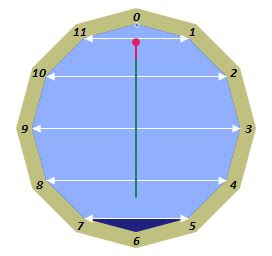AAAAA5888885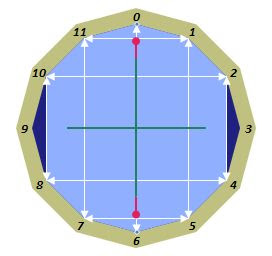988884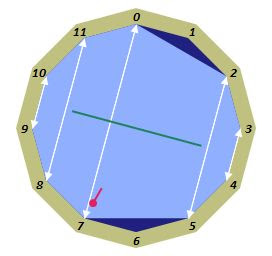666963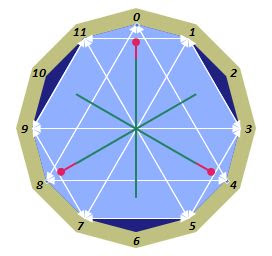876663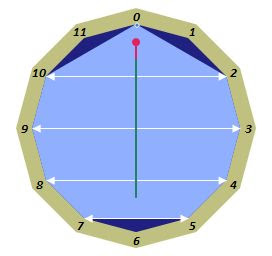448444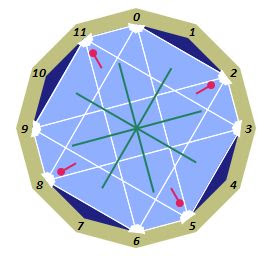765442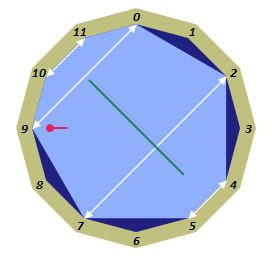254361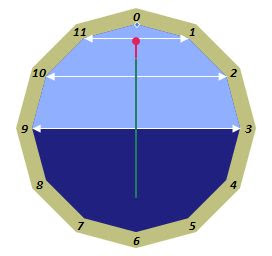654321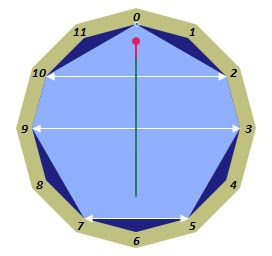060603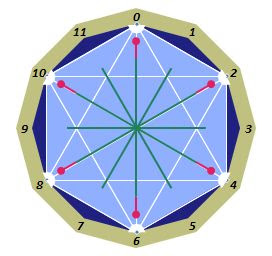543210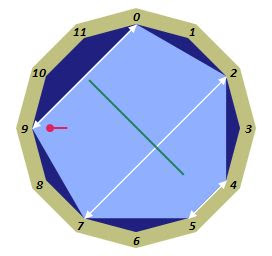032140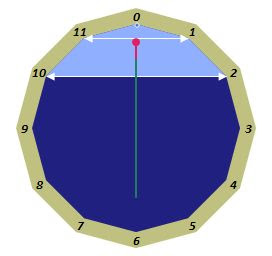432100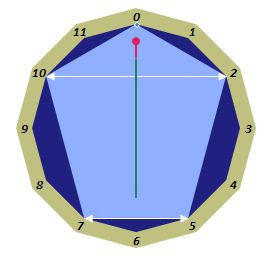004002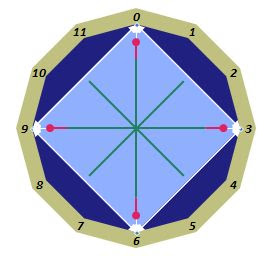321000000300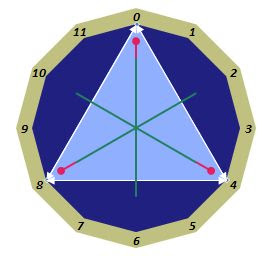210000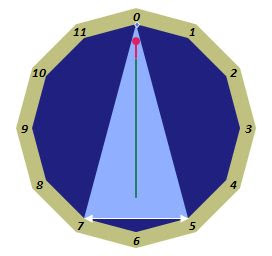000001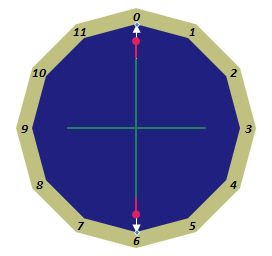100000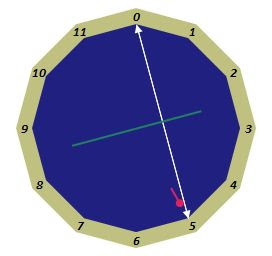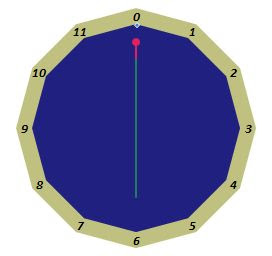000000

Basically, all we're being told here is that, to generate the scale (for a given degree) with the most possible consonances built into it, you start with the tonic (for a one note scale) and keep adding perfect fifths or fourths (i.e. intervals of class 5) to increase the scale to the next order of size. I.e. if you start with a tonic note F for a one note scale, add C to get a two note scale. With such a scale you can play the most primitive of campfire folk tunes. Now add in the fifth of the fifth (G) and we have a three note scale C F G (tonic, subdominant, dominant). Already we've got the basis for most western music. Add in G's fifth, the D, and we have a four note scale C D F G. Run and repeat that sequence quickly through your head a few times and you've got most of Philip Glass. Adding another fifth, D's A, and we have C D F G A (or the familiar five pentatonic black notes on the piano, dropped down a semitone). Bring in the A's fifth and the new E gets us the six 'best' hexatonic scales (or modes) to noodle around in, e.g. F G A C D E, or start on a D tonic if you want to be more 'minor'. Adding in the E's fifth, the B, presents us with all the white notes in all their glory C D E F G A B.

There is another re-ordering of Forte's interval vector due to Hanson who proposed that the counts of interval class frequencies should be ordered first by IV, then III, iii, II, ii and tritones (which is to say interval class set ordering 5, 4, 3, 2, 1, 6 - where our order is 5, 2, 3, 4, 1, 6 and the Fortean is 1, 2, 3, 4, 5, 6). Although the attraction of the implied 'harmoniousness' of this other scheme is evident, we still prefer ours. This is not simply for narcissistic reasons but because ours sorts to the top those scales (or chords, or pitch class sets) one would expect to see at the top (i.e. via construction from stacking Vs - or equivalently IVs - described above). Hansonian ordering, used to sort pitch class sets by alphabetised vector, would result in diatonic and pentatonic (and all similarly constructed-by-fifths sets) scales being somewhat more opaquely buried within their respective lists of pitch class set cardinalities. All three orderings are - by the way - equivalently computationally efficient, autocorrelationally speaking - Forte counts interval class frequencies straight from 1 to 11, Hanson counts from 5 to 30 - modulo 6 - in 6 steps of 5 with implicit count doubling, and we count from 7 to 77 - modulo 12, in steps of 7. Interval classwise they count exactly the same frequencies but in different orders.